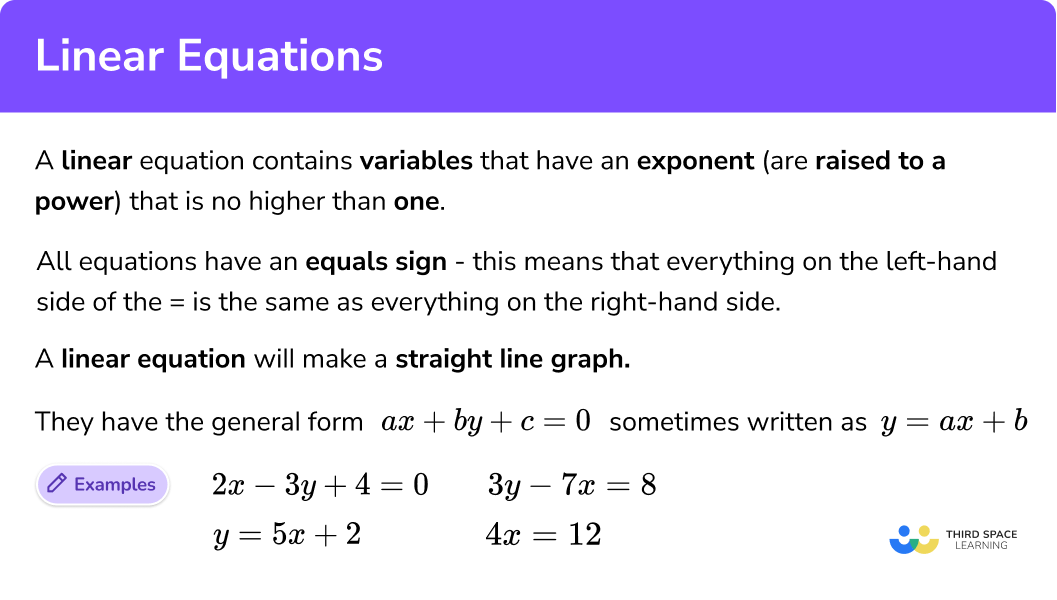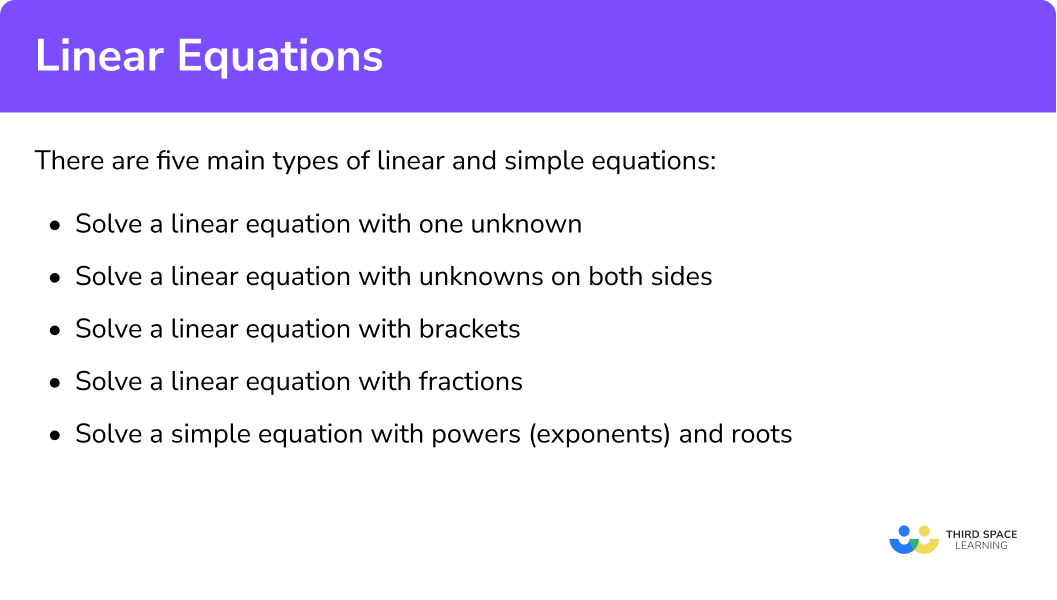# Linear Equations

Here we will learn how to solve linear and simple equations, including equations with one unknown, equations with an unknown on both sides, equations with brackets and equations with fractions.

There are also solving linear equations worksheets based on Edexcel, AQA and OCR exam questions, along with further guidance on where to go next if you’re still stuck.

## What is a linear equation?

A linear equation is an equation that contains variables that have an exponent (are raised to a power) that is no higher than one.

All equations have an equals sign, which means that everything on the left-hand side of the = is exactly the same as everything on the right-hand side.

A linear equation will make a straight line graph and have a general form of ax + by + c = 0.

This is often written as y = ax + b.

For example,

\begin{aligned} 2x-3y+4&=0\\ \\ y&=5x+2\\ \\ 3y-7x&=8\\ \\ 4x&=12 \end{aligned}

### What are linear equations?## How to solve linear equations and simple equations

We solve a linear equation by combining like terms and simplifying.

There are five main types of linear and simple equations:

a Solve linear equations with one unknown

b Solve linear equations with an unknown on both sides

c Solve linear equations with brackets

d Solve linear equations with fractions

e Solve simple equations with powers (exponents) and roots

In order to solve a linear equation or a simple equation we need to work out the value of the unknown variable by doing the opposite of what the operation tells us to do.

Top tip:
Leave the variable alone for as long as possible and deal with everything else first.

• If the equation has an addition, to ‘undo it’ we need to use subtraction

• If the equation has a subtraction, to ‘undo it’ we need to use addition

• If the equation has a multiplication, to ‘undo it’ we need to use division

• If the equation has a division, to ‘undo it’ we need to use multiplication

We can check that our answer is correct by substituting it back into the original equation.

### What are the 5 main types of linear and simple equations?### Related lessons on solving equations

Linear equations is part of our series of lessons to support revision on solving equations. You may find it helpful to start with the main solving equations lesson for a summary of what to expect, or use the step by step guides below for further detail on individual topics. Other lessons in this series include:

### a) Solve linear equations with one unknown

In order to solve  linear equations with one unknown:

1. Rearrange the equation so the unknown variable is on its own on one side
2. Work out the unknown variable by doing the opposite of what it says

To solve

$3x + 6 = 18$

we need to:

1 Rearrange the equation so the unknown variable (x) is on its own on one side

Here the opposite of +6 is −6.

2 Work out what the unknown variable (x) is by doing the opposite of what it says.

Here

$3x=3\times x$

so we divide by 3.

We can check that our solution is correct by substituting it into the original equation

### b) Solve linear equations with an unknown on both sides

In order to solve  linear equations with an unknown on both sides:

1. Rearrange the equation so the unknown variables are on the same side
2. Rearrange the equation so the unknown variable is on its own on one side
3. Work out what the unknown variable (x) is by doing the opposite of what it says

To solve

$5x + 6 = 2x + 9$

we need to:

1 Rearrange the equation so the unknown variables (x) are on the same side. (Top tip: eliminate the smallest variable).

Here 2x is smaller that 5x, so eliminate it by -2x.

2 Rearrange the equation so the unknown variable (x) is on its own on one side (here we are subtracting 6 from both sides of the equation).

3 Work out what the unknown variable (x) is by doing the opposite of what it says.

Here

$3x=3\times x$

so we divide by 3.

We can check that our solution is correct by substituting it into the original equation

### c) Solve linear equations with brackets

In order to solve  linear equations with brackets:

1. Expand the brackets
2. Solve the equation by rearranging to get the unknown variable by itself on one side and then doing the opposite of what it says.

To solve

$4(x – 2) = 12$

we need to:

1 Expand the brackets

\begin{aligned} 4(x-2) &=12 \\\\ 4 x-8 &=12 \end{aligned}

2 Solve the equation by rearranging to get the unknown variable (x) by itself on one side and then doing the opposite of what it says.

Here the opposite of −8 is +8.

Then

$4x=4\times x$

so we divide by 4.

We can check that our solution is correct by substituting it into the original equation.

Top Tip:
We could have first divided both sides of the equation by 4 for this question as 4 is a factor of 12.

### d) Solve linear equations with fractions

In order to solve linear equations with fractions:

1. Multiply each fraction by the denominator on the other side of the = to get rid of the fractions; don’t forget to include brackets.
2. Expand the brackets
3. Rearrange the equation so the unknown variables are on the same side.
4. Solve the equation by rearranging to get the unknown variable by itself on one side and then do the opposite of what it says.

To solve

$\frac{5 x-2}{4}=\frac{2 x+2}{2}$

we need to:

1 Multiply each fraction by the denominator on the other side of the = to get rid of the fractions; don’t forget to include brackets.

2 Expand the bracket

$\begin{array}{l} 2(5 x-2)=4(2 x+2) \\ 10 x-4=8 x+8 \end{array}$

3 Rearrange the equation so the unknown variables (x) are on the same side. (Top tip: eliminate the smallest variable).

Here

8x is smaller than 10x so eliminate it by -8x from both sides of the equation.

4 Solve the equation by rearranging to get the unknown variable (x) by itself on one side and then do the opposite of what it says.

We can check that our solution is correct by substituting it into the original equation.

### e) Solve simple equations with powers and roots

In order to solve simple equations with powers and roots:

1. Rearrange the equation so the unknown variable is on its own on one side
2. Work out the unknown variable by doing the opposite of what it says

To solve

$4x^{2}=64$

we need to:

1 Rearrange to get the unknown variable (x2) by itself on one side.

Here

$4x^{2}=4\times x^{2}$

so we divide by 4.

2 Work out the unknown variable by doing the opposite of what it says.

The opposite of squaring is to take the square root, so do this to both sides.

(Remember square roots have a positive and a negative answer)

To solve

$5\sqrt{x}=30$

we need to:

1 Rearrange to get the unknown variable (√x) by itself on one side.

Here

$5\sqrt{x}=5\times \sqrt{x}$

so we divide by 5.

2 Work out the unknown variable by doing the opposite of what it says.

The opposite of taking the square root is squaring, so do this to both sides.

We can check that our solution is correct by substituting it into the original equation.

### Common misconceptions

• We must do the opposite of what the operation tells us to do

E.g.

3x = 12

means 3 × x = 12. So to solve the equation for x we have to do the opposite.

The opposite of × 3 is ÷ 3.

E.g.

$\frac{x}{2}=4$

means x ÷ 2 = 4. So to solve the equation for x we have to do the opposite.
The opposite of ÷ 2 is × 2

• We must do the same thing to both sides of the equal sign

Equals means that both sides of the = are exactly the same.

E.g.

If we −2 on the left of the equal sign, we have to −2 on the right of the equal sign

• When cross multiplying we must use brackets to multiply every term in the numerator

We only multiply the numerator, not the denominator.

E.g.

• When expanding brackets we need to multiply out each term

E.g.

$\quad 2(6x-3)=12x-6$ $2\times 6x=12 \quad \quad 2\times -3=-6$

Be careful when multiplying the coefficients

• Remember a polynomial is an algebraic expression that consists of two or more algebraic terms

### Practice linear equations questions

1. Solve:

2x+8=20

x=14x=8x=6x=102x+8=20

Subtract 8 from both sides

2x=12

Divide both sides by 2

x=6

2. Solve:

5x-3=12

x=5x=-8x=3x=-125x-3=12

5x=15

Divide both sides by 5

x=3

3. Solve:

2x-7=x+5

x=7x=12x=-7x=42x-7=x+5

Subtract x from both sides

x-7=5

x=12

4. Solve:

7x-3=4x+15

x=4x=7x=6x=37x-3=4x+15

Subtract 4x from both sides

3x-3=15

3x=18

Divide both sides by 3

x=6

5. Solve:

5(x-3)=10

x=2x=3x=5x=-35(x-3)=10

Divide both sides by 5

x-3=2

x=5

6. Solve:

3(x-3)=6(x+5)

x=3x=-5x=-13x=133(x-3)=6(x+5)

Expand the brackets

3x-9=6x+30

Subtract 3x from both sides

-9=3x+30

Subtract 30 from both sides

-39=3x

Divide both sides by 3

x=-13

7. Solve:

\frac{x+3}{2}=\frac{x+4}{3}

x=-3x=-4x=-2x=-1\frac{x+3}{2}=\frac{x+4}{3}

Multiply both sides by 6 and simplify

3x+9=2x+8

From both sides, subtract 2x and subtract 9

x=-1

8. Solve:

\frac{3x+1}{2}+\frac{2x-2}{4}

x=-2x=2x=-1x=1\frac{3x+1}{2}+\frac{2x-2}{4}

Multiply both sides by 4 and simplify

6x+2=2x-2

From both sides, subtract 2x and subtract 2

x=-1

9. Solve:

16{x}^2=64

x=\pm4x=\pm2x=\pm3x=\pm1616{x}^2=64

Divide both sides by 16

x^{2}=4

Square root both sides

x=\pm2

10. Solve:

5\sqrt{3x}=30

x=36x=3x=12x=95\sqrt{3x}=30

Divide both sides by 5

\sqrt{3x}=6

Square both sides

3x=36

Divide both sides by 3

x=12

### Linear equations GCSE questions

1. Solve:

3 x^{2} = 27

(1 mark)

x=\pm 3

(1)

2. Solve:

4(3 – x) = 32

(2 marks)

12 – 4x = 32  or  3 – x = 8

(1)

x = -5

(1)

3. Solve:

\frac{2x + 3}{5}=\frac{x – 5}{2}

(3 marks)

2(2x + 3) = 5(x – 5)

(1)

4x + 6 = 5x – 25

(1)

x=31

(1)

## Learning checklist

You have now learned how to:

• Use algebraic methods to solve linear equations.

## Still stuck?

Prepare your KS4 students for maths GCSEs success with Third Space Learning. Weekly online one to one GCSE maths revision lessons delivered by expert maths tutors.

Find out more about our GCSE maths tuition programme.